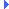FD.io VPP  v18.07.1-13-g909ba93 Vector Packet Processing
svm_fifo_segment.c File ReferenceInclude dependency graph for svm_fifo_segment.c:

Go to the source code of this file.

## Functions

static void allocate_new_fifo_chunk (svm_fifo_segment_header_t *fsh, u32 data_size_in_bytes, int chunk_size)

void svm_fifo_segment_preallocate_fifo_pairs (svm_fifo_segment_private_t *s, u32 rx_fifo_size, u32 tx_fifo_size, u32 *n_fifo_pairs)
Pre-allocates fifo pairs in fifo segment. More...

int svm_fifo_segment_init (svm_fifo_segment_private_t *s)
Initialize svm fifo segment shared header. More...

int svm_fifo_segment_create (svm_fifo_segment_create_args_t *a)
Create an svm fifo segment and initialize as master. More...

int svm_fifo_segment_create_process_private (svm_fifo_segment_create_args_t *a)
Create an svm fifo segment in process-private memory. More...

int svm_fifo_segment_attach (svm_fifo_segment_create_args_t *a)
Attach as slave to an svm fifo segment. More...

void svm_fifo_segment_delete (svm_fifo_segment_private_t *s)

svm_fifo_tsvm_fifo_segment_alloc_fifo (svm_fifo_segment_private_t *s, u32 data_size_in_bytes, svm_fifo_segment_freelist_t list_index)
Allocate fifo in svm segment. More...

void svm_fifo_segment_free_fifo (svm_fifo_segment_private_t *s, svm_fifo_t *f, svm_fifo_segment_freelist_t list_index)

void svm_fifo_segment_main_init (u64 baseva, u32 timeout_in_seconds)

u32 svm_fifo_segment_index (svm_fifo_segment_private_t *s)

svm_fifo_segment_private_tsvm_fifo_segment_segments_pool (void)
Retrieve svm segments pool. More...

u32 svm_fifo_segment_num_fifos (svm_fifo_segment_private_t *fifo_segment)
Get number of active fifos. More...

u32 svm_fifo_segment_num_free_fifos (svm_fifo_segment_private_t *fifo_segment, u32 fifo_size_in_bytes)

void svm_fifo_segment_info (svm_fifo_segment_private_t *seg, uword *address, u64 *size)

mheap_tsvm_fifo_segment_heap (svm_fifo_segment_private_t *seg)

u8format_svm_fifo_segment_type (u8 *s, va_list *args)

u8format_svm_fifo_segment (u8 *s, va_list *args)
Segment format function. More...

## Variables

svm_fifo_segment_main_t svm_fifo_segment_main

## Function Documentation

 static void allocate_new_fifo_chunk ( svm_fifo_segment_header_t * fsh, u32 data_size_in_bytes, int chunk_size )
static

Definition at line 21 of file svm_fifo_segment.c.Here is the call graph for this function:Here is the caller graph for this function:

 u8* format_svm_fifo_segment ( u8 * s, va_list * args )

Segment format function.

Definition at line 616 of file svm_fifo_segment.c.Here is the call graph for this function:

 u8* format_svm_fifo_segment_type ( u8 * s, va_list * args )

Definition at line 595 of file svm_fifo_segment.c.Here is the call graph for this function:

 svm_fifo_t* svm_fifo_segment_alloc_fifo ( svm_fifo_segment_private_t * s, u32 data_size_in_bytes, svm_fifo_segment_freelist_t list_index )

Allocate fifo in svm segment.

Definition at line 329 of file svm_fifo_segment.c.Here is the call graph for this function:Here is the caller graph for this function:

 int svm_fifo_segment_attach ( svm_fifo_segment_create_args_t * a )

Attach as slave to an svm fifo segment.

Definition at line 283 of file svm_fifo_segment.c.Here is the call graph for this function:Here is the caller graph for this function:

 int svm_fifo_segment_create ( svm_fifo_segment_create_args_t * a )

Create an svm fifo segment and initialize as master.

Definition at line 201 of file svm_fifo_segment.c.Here is the call graph for this function:Here is the caller graph for this function:

 int svm_fifo_segment_create_process_private ( svm_fifo_segment_create_args_t * a )

Create an svm fifo segment in process-private memory.

Definition at line 235 of file svm_fifo_segment.c.Here is the call graph for this function:Here is the caller graph for this function:

 void svm_fifo_segment_delete ( svm_fifo_segment_private_t * s )

Definition at line 316 of file svm_fifo_segment.c.Here is the call graph for this function:Here is the caller graph for this function:

 void svm_fifo_segment_free_fifo ( svm_fifo_segment_private_t * s, svm_fifo_t * f, svm_fifo_segment_freelist_t list_index )

Definition at line 426 of file svm_fifo_segment.c.Here is the call graph for this function:Here is the caller graph for this function:

 mheap_t* svm_fifo_segment_heap ( svm_fifo_segment_private_t * seg )

Definition at line 589 of file svm_fifo_segment.c.Here is the caller graph for this function:

 u32 svm_fifo_segment_index ( svm_fifo_segment_private_t * s )

Definition at line 495 of file svm_fifo_segment.c.Here is the caller graph for this function:

 void svm_fifo_segment_info ( svm_fifo_segment_private_t * seg, uword * address, u64 * size )

Definition at line 570 of file svm_fifo_segment.c.Here is the call graph for this function:Here is the caller graph for this function:

 int svm_fifo_segment_init ( svm_fifo_segment_private_t * s )

Initialize svm fifo segment shared header.

Definition at line 178 of file svm_fifo_segment.c.Here is the call graph for this function:Here is the caller graph for this function:

 void svm_fifo_segment_main_init ( u64 baseva, u32 timeout_in_seconds )

Definition at line 486 of file svm_fifo_segment.c.Here is the caller graph for this function:

 u32 svm_fifo_segment_num_fifos ( svm_fifo_segment_private_t * fifo_segment )

Get number of active fifos.

Definition at line 514 of file svm_fifo_segment.c.Here is the caller graph for this function:

 u32 svm_fifo_segment_num_free_fifos ( svm_fifo_segment_private_t * fifo_segment, u32 fifo_size_in_bytes )

Definition at line 520 of file svm_fifo_segment.c.Here is the call graph for this function:Here is the caller graph for this function:

 void svm_fifo_segment_preallocate_fifo_pairs ( svm_fifo_segment_private_t * s, u32 rx_fifo_size, u32 tx_fifo_size, u32 * n_fifo_pairs )

Pre-allocates fifo pairs in fifo segment.

The number of fifos pre-allocated is the minimum of the requested number of pairs and the maximum number that fit within the segment. If the maximum is hit, the number of fifo pairs requested is updated by subtracting the number of fifos that have been successfully allocated.

Definition at line 68 of file svm_fifo_segment.c.Here is the call graph for this function:Here is the caller graph for this function:

 svm_fifo_segment_private_t* svm_fifo_segment_segments_pool ( void )

Retrieve svm segments pool.

Used only for debug purposes.

Definition at line 504 of file svm_fifo_segment.c.Here is the caller graph for this function:

## Variable Documentation

 svm_fifo_segment_main_t svm_fifo_segment_main

Definition at line 18 of file svm_fifo_segment.c.